Open in App
Not now

# Solve Differential Equations with ODEINT Function of SciPy module in Python

• Last Updated : 09 Oct, 2022

In this post, we are going to learn how to solve differential equations with odeint function of scipy module in Python.

ODE stands for Ordinary Differential Equation and refers to those kinds of differential equations that involve derivatives but no partial derivatives. In other words, we only consider one independent variable in these equations.

So, in this article we have used scipy, NumPy, and Matplotlib modules of python which you can install with the following command:

`pip install scipy numpy matplotlib`

The syntax of odeint functions is as follows:

odeint(func, y0, t, …..)

Parameters :
model– the differential equation
y0– Initial value of Y
t– the time space for which we want the curve(basically the range of x)

A lot more other parameters are there which you can check out in the documentation but these three parameters are the main ones.

## Solving Differential Equations

Now let us solve some ODE with the help of the odeint function.

### Example 1: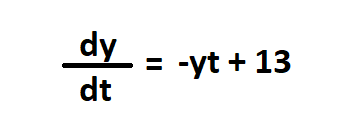Ordinary Differential Equation

## Python3

 `import` `numpy as np ` `from` `scipy.integrate ``import` `odeint ` `import` `matplotlib.pyplot as plt ` ` `  `def` `returns_dydt(y,t): ` `    ``dydt ``=` `-``y ``*` `t ``+` `13` `    ``return` `dydt ` ` `  `# initial condition ` `y0 ``=` `1` ` `  `# values of time ` `t ``=` `np.linspace(``0``,``5``) ` ` `  `# solving ODE ` `y ``=` `odeint(returns_dydt, y0, t) ` ` `  `# plot results ` `plt.plot(t,y) ` `plt.xlabel(``"Time"``) ` `plt.ylabel(``"Y"``) ` `plt.show()`

Output: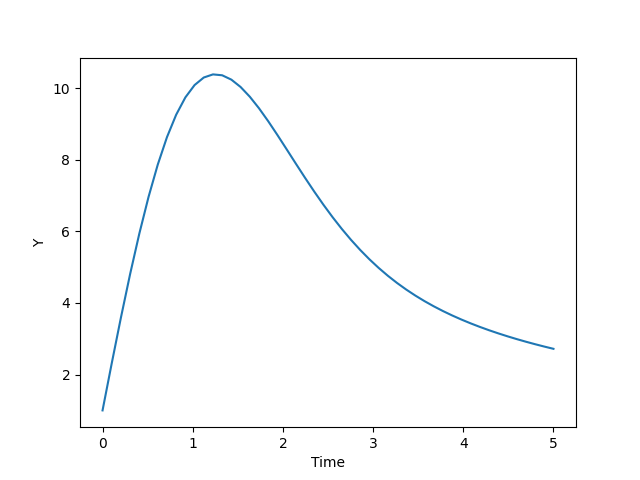Graph for the solution of ODE

### Example 2: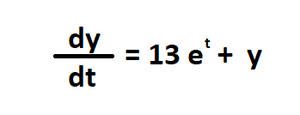Ordinary Differential Equation

## Python3

 `import` `numpy as np ` `from` `scipy.integrate ``import` `odeint ` `import` `matplotlib.pyplot as plt ` ` `  `def` `returns_dydt(y,t): ` `    ``dydt ``=` `13` `*` `np.exp(t) ``+` `y ` `    ``return` `dydt ` ` `  `# initial condition ` `y0 ``=` `1` ` `  `# values of time ` `t ``=` `np.linspace(``0``,``5``) ` ` `  `# solving ODE ` `y ``=` `odeint(returns_dydt, y0, t) ` ` `  `# plot results ` `plt.plot(t,y) ` `plt.xlabel(``"Time"``) ` `plt.ylabel(``"Y"``) ` `plt.show()`

Output: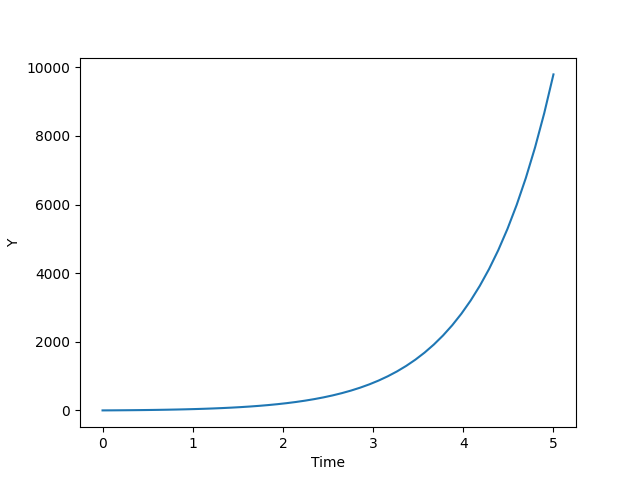Graph for the solution of ODE

### Example 3: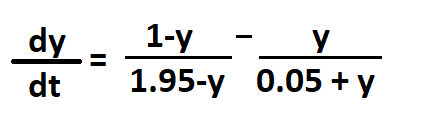Ordinary Differential Equation

Let us try giving multiple initial conditions by making y0 an array.

## Python3

 `import` `numpy as np ` `from` `scipy.integrate ``import` `odeint ` `import` `matplotlib.pyplot as plt ` ` `  `def` `returns_dydt(y,t): ` `    ``dydt ``=` `(``1``-``y)``/``(``1.95``-``y) ``-` `y``/``(``0.05``+``y) ` `    ``return` `dydt ` ` `  `# initial conditions ` `y0 ``=` `[``0``, ``1``, ``2``] ` ` `  `# values of time ` `t ``=` `np.linspace(``1``,``10``) ` ` `  `# solving ODE ` `y ``=` `odeint(returns_dydt, y0, t) ` ` `  `# plot results ` `plt.plot(t,y) ` `plt.xlabel(``"Time"``) ` `plt.ylabel(``"Y"``) ` `plt.show()`

Output: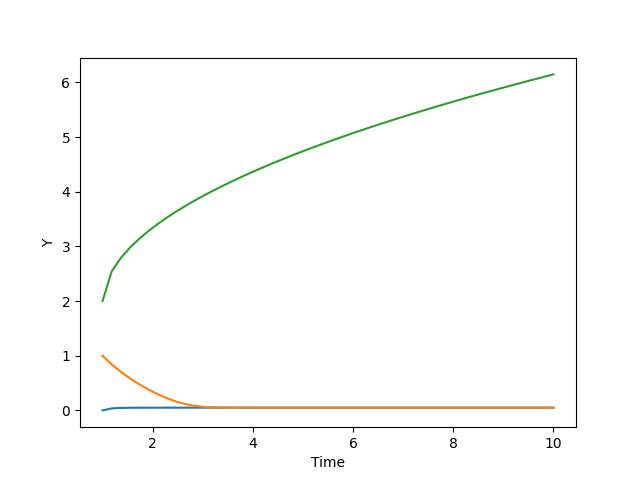Graph for the solution of ODE

My Personal Notes arrow_drop_up
Related Articles